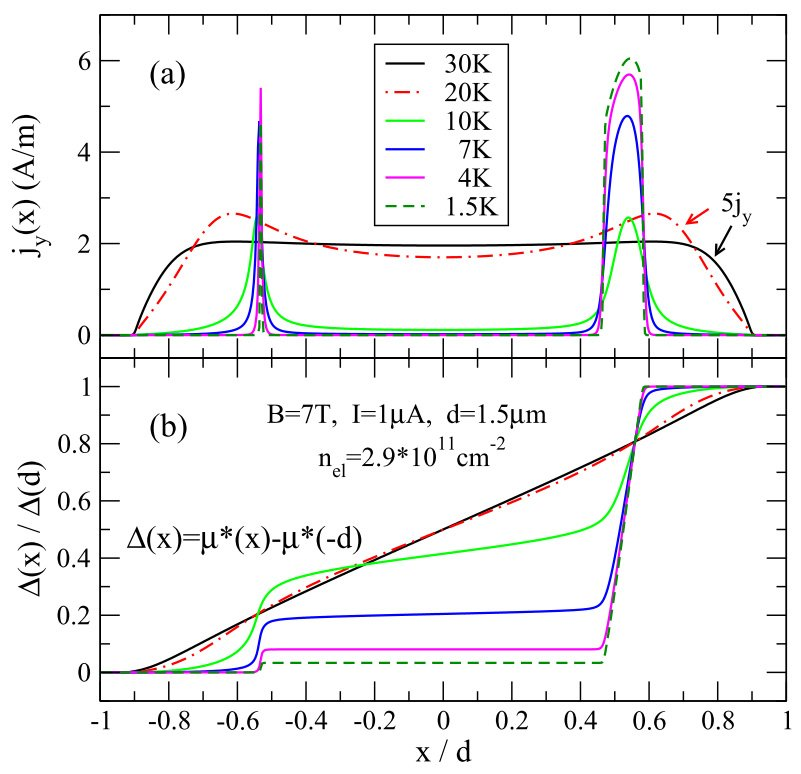# Screening and transport in narrow quantum Hall systems

As a consequence of the singular Landau density of states, screening in an inhomogeneous two-dimensional electron system (2DES) subject to a strong perpendicular magnetic ﬁeld B is, at low temperature T, extremely nonlinear. The system splits into "compressible" regions, in which screening is nearly perfect and the electron distribution arranges so that, within kBT, a Landau level is pinned to the electrochemical potential µ*, and into "incompressible" regions in between, in which µ* falls into a gap between adjacent Landau levels, so that there the Landau-level ﬁlling factor ν has a ﬁxed integer value and, therefore, the electron density is constant [1-3]. Within an incompressible strip (IS) no elastic scattering is possible (since there are no states at the Fermi level), so that the longitudinal and the Hall resistivity there have the values of the free electron system with the same integer ﬁlling factor, i.e., in the limit T → 0 results ρ = 0 and ρH = h/(νe2), respectively. In a narrow Hall bar, where the 2D electron density decreases monotonously from a maximum in the center to zero at the edges, ISs of ﬁnite width exist in certain intervals of the magnetic ﬁeld B. If an imposed current leads to a stationary non-equilibrium state of the 2DES, according to the thermodynamic principle of minimum entropy production the current density concentrates on these ISs, where the current ﬂows dissipationless.  A quasi-local Ohmic transport theory, which in a homogeneous 2DES describes the Shubnikov-de Haas oscillations, combined with a self-consistent screening theory, describes the integer quantized Hall effect (IQHE) in narrow Hall bars, and the plateaus of the IQHE appear as the B-intervals in which sufﬁciently wide ISs exist [4-6]. Early  and more recent scanning force microscope measurements in the group of J. Weis conﬁrm the predictions of this theory, and it is of great interest to investigate its further predictions, limitations, and possible extensions.Figure 1: (a) Current density along and (b) normalized Hall potential across a Hall bar containing a 2DES with translation invariance in y-direction and lateral conﬁnement in x-direction due to metallic gates in the half-planes z = 0, x < −d and z = 0, x > d = 1.5µm. The imposed current I = 1µA and the magnetic ﬁeld B = 7T are ﬁxed. At higher temperature, T  ≥ 30K, the current density is proportional to the electron density, and the Hall potential varies linearly across the 2DES. With decreasing T, the current density concentrates more and more around the stripes with local ﬁlling factor ν(x) = 2, where incompressible stripes (ISs) form. In the limit T → 0 all the imposed current ﬂows dissipationless through, and the total Hall voltage drops across these ISs. Nonlinear feedback leads to strong asymmetries.

References:
  D.B. Chklovskii, B.I. Shklovskii, and L.I. Glazman. Phys. Rev. B 46, 4026 (1992).
  K. Lier and R.R. Gerhardts. Phys. Rev. B 50, 7757 (1994).
  J.H. Oh and R.R. Gerhardts. Phys. Rev. B 56, 13519 (1997).
  Güven K. and R.R. Gerhardts. Phys. Rev. B 67, 115327 (2003).
  Siddiki A. and R.R. Gerhardts. Phys. Rev. B 70, 195335 (2004).
  R.R. Gerhardts. phys. stat. sol. (b) 245, 378 (2008).
  E. Ahlswede, P. Weitz, J. Weis, K. von Klitzing, and K. Eberl. Physica B 298, 562 (2001).

Go to Editor View